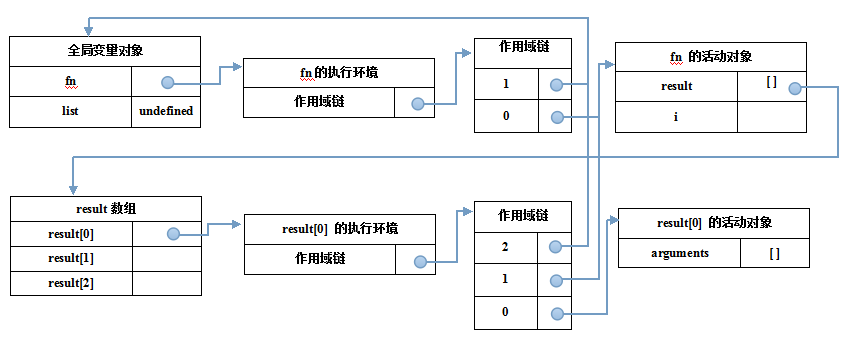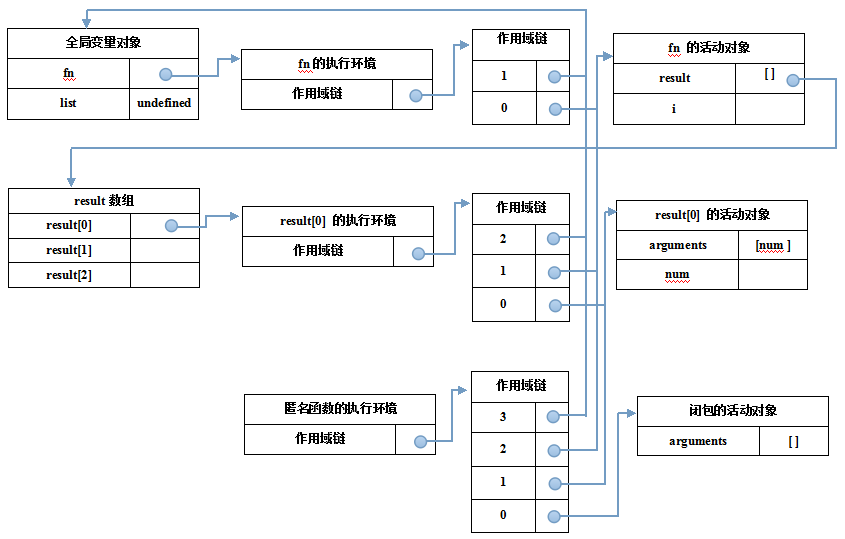JavaScript Note - Closures

Closures are functions that refer to independent (free) variables

var fn = function () {
var result = [];
for (var i = 0; i < 3; i++) {
result[i] = function () {
console.log(i);
};
}
return result;
};

var lists = ();
lists(); // 3
lists(); // 3
lists(); // 3
var fn = function () {
var result = [];
for (var i = 0; i < 3; i++) {
result[i] = (function () {
return function (num) {
console.log(num);
}
})(i);
}
return result;
};

var lists = ();
lists(); // 0
lists(); // 1
lists(); // 2function foo (a) {
return function (b) {
return a+++b;
};
}

var a = foo(10);
var b = foo(20);

var arrs = [a(10), b(20), a(10)];